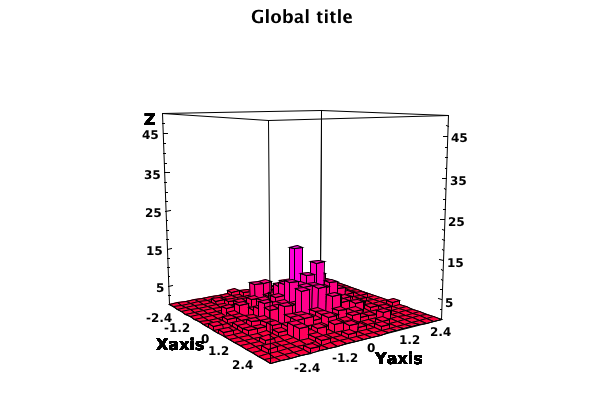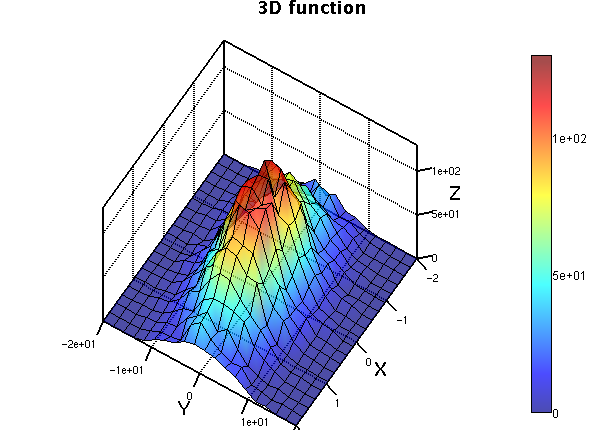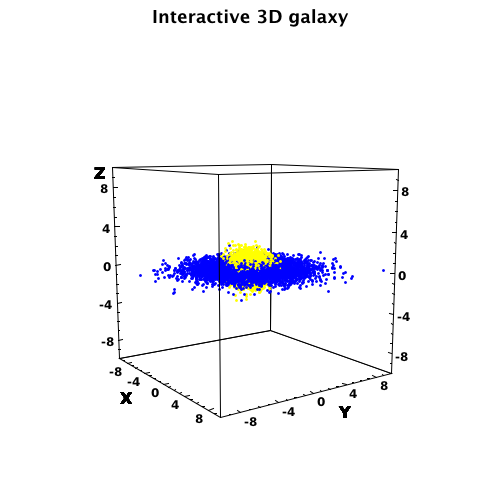# DScience:3D representation of data

Limitted access. Login to DataMelt if you are a full DataMelt member.

30% complete

3D plots use 3D computer graphics to represent scientific data. Data are usually defined as [math]Z=f(X,Y)[/math], where [math](X,Y,Z)[/math] values by a function or actual data. There are a number of possible types of 3D plots. You can use the following Java classes to plot data in 3D:jhplot.HPlot3D,jhplot.HPlotXYZ,jhplot.HPlot3DP,jhplot.HVisAd,jhplot.HPlotMX. See additional details in DMelt:Plots/5_Plots_in_3D.

## Bar plots or histograms

A bar plot draws a three-dimensional bar chart, where each element in Z corresponds to one bar. To make such plots from a 3D histogram use the Java classjhplot.H2D. Here is the Python code with an example:```from jhplot  import HPlot3D,SHPlot3D,H2D
from java.util import Random

# build a standard canvas
c1 = HPlot3D("Canvas",600,400)

# build a singleton
# c1=SHPlot3D.getCanvas()

c1.setGTitle("Global title")
c1.setNameX("Xaxis")
c1.setNameY("Yaxis")
c1.visible(1)

h1 = H2D("My 2D Test",20,-3.0, 3.0, 20, -3.0, 3.0)
rand = Random();
for i in range(500):
h1.fill(rand.nextGaussian(),rand.nextGaussian())
c1.draw(h1);

# export to some image (png,eps,pdf,jpeg...)
# c1.export(Editor.DocMasterName()+".png")```

## Surface plots

Here is an example of surface plots:## Scattered data

Here is an example that use two data points (blue and red):## Mixed plots

One can overlay scattered data with surface plots, bar plots in arbitrary way. Typically, you need to usejhplot.HPlot3D canvas.

## Example code

This tutorial is provided under this license agreement.